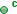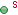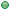# Altova MapForce 2023 Professional Edition

## com.altova.types.DateTime

This class enables you to process XML attributes or elements that have date and time types, such as xs:dateTime.

## Constructors

Name

Descriptionpublic DateTime()

Initializes a new instance of the DateTime class to an empty value.public DateTime(DateTime newvalue)

Initializes a new instance of the DateTime class to the DateTime value supplied as argument.public DateTime(int newyear, int newmonth, int newday, int newhour, int newminute, int newsecond, double newpartsecond, int newoffsetTZ)

Initializes a new instance of the DateTime class to the year, month, day, hour, minute, second, the fractional part of the second, and timezone supplied as arguments. The fractional part of the second newpartsecond must be between 0 and 1. The timezone offset newoffsetTZ can be either positive or negative and is expressed in minutes.public DateTime(int newyear, int newmonth, int newday, int newhour, int newminute, int newsecond, double newpartsecond)

Initializes a new instance of the DateTime class to the year, month, day, hour, minute, second, and the fractional part of a second supplied as arguments.public DateTime(int newyear, int newmonth, int newday)

Initializes a new instance of the DateTime class to the year, month, and day supplied as arguments.public DateTime(Calendar newvalue)

Initializes a new instance of the DateTime class to the java.util.Calendar value supplied as argument.

## Methods

Name

Descriptionstatic DateTime now()

Returns the current time as a DateTime object.static DateTime parse(String s)

Returns a DateTime object parsed from the string value supplied as argument. For example, the following sample string values would be converted successfully to a DateTime object:

2015-11-24T12:54:47.969+01:00

2015-11-24T12:54:47

2015-11-24int getDay()

Returns the day of the current DateTime instance.int getHour()

Returns the hour of the current DateTime instance.int getMillisecond()

Returns the millisecond of the current DateTime instance, as an integer value.int getMinute()

Returns the minute of the current DateTime instance.int getMonth()

Returns the month of the current DateTime instance.double getPartSecond()

Returns the fractional part of the second of the current DateTime instance, as a double value. The return value is greater than zero and smaller than one, for example:

0.313int getSecond()

Returns the second of the current DateTime instance.int getTimezoneOffset()

Returns the timezone offset, in minutes, of the current DateTime instance. For example, the timezone "UTC-01:00" would be returned as:

-60Calendar getValue()

Returns the current DateTime instance as a java.util.Calendar value.int getWeekday()

Returns the day in week of the current DateTime instance. Values range from 0 through 6, where 0 is Monday (ISO-8601).int getYear()

Returns the year of the current DateTime instance.int hasTimezone()

Returns information about the timezone of the current DateTime instance. Possible return values are:

 CalendarBase.TZ_MISSING A timezone offset is not defined. CalendarBase.TZ_UTC The timezone is UTC. CalendarBase.TZ_OFFSET A timezone offset has been defined.void setDay( int nDay )

Sets the day of the current DateTime instance to the value supplied as argument.void setHasTimezone( int nHasTZ )

Sets the timezone information of the current DateTime instance to the value supplied as argument. This method can be used to strip the timezone information or set the timezone to UTC (Coordinated Universal Time). Valid values for the nHasTZ argument:

 CalendarBase.TZ_MISSING Set the timezone offset to undefined. CalendarBase.TZ_UTC Set the timezone to UTC. CalendarBase.TZ_OFFSET If the current object has a timezone offset, leave it unchanged.void setHour( int nHour )

Sets the hour of the current DateTime instance to the value supplied as argument.void setMinute( int nMinute )

Sets the minute of the current DateTime instance to the value supplied as argument.void setMonth( int nMonth )

Sets the month of the current DateTime instance to the value supplied as argument.void setPartSecond( double nPartSecond )

Sets the fractional part of the second of the current DateTime instance to the value supplied as argument.void setSecond( int nSecond )

Sets the second of the current DateTime instance to the value supplied as argument.void setTimezoneOffset( int nOffsetTZ )

Sets the timezone offset of the current DateTime instance to the value supplied as argument. The value nOffsetTZ must be an integer (positive or negative) and must be expressed in minutes.void setYear( int nYear )

Sets the year of the current DateTime instance to the value supplied as argument.String toString()

Returns the string representation of the current DateTime instance, for example:

2015-11-24T15:50:56.968+01:00

## Examples

Before using the following code listings in your program, ensure the Altova types are imported:

 import com.altova.types.*;

The following code listing illustrates various ways to create DateTime objects:

 protected static void DateTimeExample1(){   // Initialize a new instance of the DateTime class to the current time   DateTime dt = new DateTime(DateTime.now());   System.out.println("DateTime created from current date and time: " + dt.toString());         // Initialize a new instance of the DateTime class by supplying the parts   DateTime dt1 = new DateTime(2015, 11, 23, 14, 30, 24, .459);   System.out.println("DateTime from parts (no timezone): " + dt1.toString());    // Initialize a new instance of the DateTime class by supplying the parts   DateTime dt2 = new DateTime(2015, 11, 24, 14, 30, 24, .459, -60);   System.out.println("DateTime from parts (with negative timezone): " + dt2.toString());         // Initialize a new instance of the DateTime class by parsing a string value           DateTime dt3 = DateTime.parse("2015-11-24T12:54:47.969+01:00");             System.out.println("DateTime parsed from string: " + dt3.toString());         }

The following code listing illustrates getting values from DateTime objects:

 protected static void DateTimeExample2()   {     // Initialize a new instance of the DateTime class to the current time     DateTime dt = new DateTime(DateTime.now());             // Output the formatted year, month, and day of this DateTime instance     String str1 = String.format("Year: %d; Month: %d; Day: %d;", dt.getYear(), dt.getMonth(), dt.getDay());     System.out.println(str1);             // Output the formatted hour, minute, and second of this DateTime instance     String str2 = String.format("Hour: %d; Minute: %d; Second: %d;", dt.getHour(), dt.getMinute(), dt.getSecond());     System.out.println(str2);             // Return the timezone (in minutes) of this DateTime instance     System.out.println("Timezone: " + dt.getTimezoneOffset());             // Get the DateTime as a java.util.Calendar value     java.util.Calendar dt_java = dt.getValue();     System.out.println("" + dt_java.toString());             // Return the day of week of this DateTime instance     System.out.println("Weekday: " + dt.getWeekday());                   // Check whether the DateTime instance has a timezone defined     switch(dt.hasTimezone())     {         case CalendarBase.TZ_MISSING:           System.out.println("No timezone.");           break;         case CalendarBase.TZ_UTC:                       System.out.println("The timezone is UTC.");           break;         case CalendarBase.TZ_OFFSET:           System.out.println("This object has a timezone.");           break;         default:           System.out.println("Unable to determine whether a timezone is defined.");           break;     }         }

The following code listing illustrates changing the timezone offset of a DateTime object:

 protected static void DateTimeExample3(){         // Create a new DateTime object with timezone -0100 UTC   DateTime dt = new DateTime(2015, 11, 24, 14, 30, 24, .459, -60);   // Output the value before the change   System.out.println("Before: " + dt.toString());   // Change the offset to +0100 UTC   dt.setTimezoneOffset(60);               // Output the value after the change   System.out.println("After:  " + dt.toString()); }